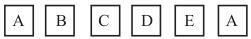# A child has a die whose six faces show the letters as given below: The die is thrown once. What is the probability of getting (i) A? (ii) D?The total number of possible outcomes (or events) = 6

P(E) = (Number of favourable outcomes/ Total number of outcomes)

(i) The total number of faces having A on it = 2

P (getting A) = 2/6 = ⅓ = 0.33

(ii) The total number of faces having D on it = 1

P (getting D) = ⅙ = 0.166